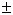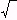edHelper subscribers - Create a new printable
Answer key also includes questions
Answer key only gives the answers
No answer key

Not a subscriber?  Sign up now for the subscriber materials!
Sample edHelper.com - QuadraticEquations Worksheet
Return to Quadratic Equation Worksheets
Return to Algebra Worksheets
Return to Math

 Name _____________________________Date ___________________
Quadratic Equations
(Answer ID # 0718163)
Write a quadratic equation that has the given roots.

1.
417

2.
 { 1 , -3 }

3.
 { 11 , -1 }

4.
 { 1 , -8 }

5.
 1i3

6.
 -2i3

7.
1173

8

8.
 { 2 , 1 }

9.
1
 38
,  -1 }

10.
1  ,
-1
 37
}

11.
 { -2 , -4 }

12.
 3i10

13.
 26i5

14.
-197

24

15.
-1  ,
-2
 23
}

 Answer Key

Sample
This is only a sample worksheet.

edHelper subscribers - Create a new printable
Answer key also includes questions
Answer key only gives the answers
No answer key

Not a subscriber?  Sign up now for the subscriber materials!
Sample edHelper.com - QuadraticEquations Worksheet
Return to Quadratic Equation Worksheets
Return to Algebra Worksheets
Return to Math